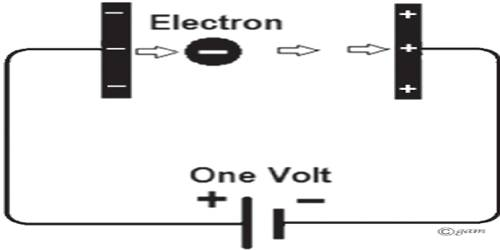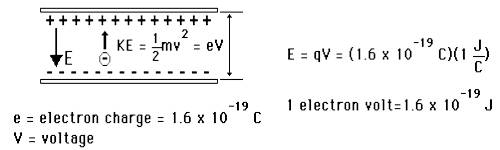Physics

# Electron VoltElectron volt: In atomic and nuclear physics work or energy is often measured instead of joule, by another quantity called electron volt. If the potential difference between two points in an electric field is 1V, then the kinetic energy acquired by a free electron in moving from one point to another point is called 1 electron volt or simply 1 eV.

So, 1 eV = charge of an electron x 1 volt.

= 1.6 x 10-19 C x 1V = 1.6 x 10-19 C x (1J/1C) = 1.6 x 10-19 [1V = (1J/1C)]

Definition: The amount of work done in taking a free electron from a point to another point having potential difference of 1V is called 1 electron volt (1 eV).It is a unit of energy equal to the energy gained by an electron in passing from a point of low potential to a point one volt higher in potential: 1.60 × 10-19 joule.

It is a very small unit. So, in physics MeV (Mega electron volt), BeV (Beta electron volt) or, GeV (Gega election volt) are used extensively.

1 MeV = 106 eV; 1 BeV = 109 eV; 1 GeV = 1012 eV.